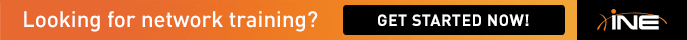# OSPF study references

Why don't we gather each candidate's unique study reference,

and share them among us?

More efficient than self study.

OSPF Areas

http://www.mav.kiev.ua/cisco/pix/lsa_diag.png

OSPF Opening Moves

http://www.netmasterclass.com/images/misc/COD-IGP/igp_block2.gif

• Hi Sakamoto,

What type of reference you are talking about, about CCIE exam or else? Please make it clear.

HTH

•

In OSPF field, we gather OSPF.

In BGP, BGP.

• Yes, we can have discussion on the same without violating NDA because we can't disclose anything that we have seen in the real lab exam [:)]

•

(PPT)

Configuring the Open Shortest Path First Protocol

•

OSPF Basics 10 July 2013

OSPF Basics

APNIC is giving us free lectures.

•

OSPF Bandwidth Calculator

http://www.cc-consult.co.uk/ospfcalc.htm

• OSPF Bandwidth Calculator

No ospf calculators are allowed in the lab. We must memorize the formula, because  looking it up in the lab will take up time.  It is much easier than EIGRP.

referenceBW/BW = cost

*default referenceBW = 100,000,000 or 100Mb

• It is much easier than EIGRP

In fact you don't need to memorise the EIGRP formula!  show ip eigrp topology x.x.x.x will give you all the information that you need.

• In fact you don't need to memorise the EIGRP formula!  show ip eigrp topology x.x.x.x will give you all the information that you need.

Hi welshy,

The K-values are there, which is nice to know.   But if we need to quickly calculate for variable paths, shouldn't we use the formula?

I remember there being a really good task in the EIGRP section (vol-I).   Since I am starting to work on speed, I found that it is a bit slow to just keep changing metrics to get the correct required ratio.

Please let me know if you know of a good routine to calculate ratios for variable paths (EIGRP).  Any help is appreciated.  Thanks.

Default EIGRP Metric =     256*(Bandwidth + totalDelay)

*bandwidth = 10^7/minBW

Long Version:

[ K1 * bandwidth + (K2 * bandwidth)/256 - load) + K3 * delay] * [ K5/(reliability + K4)]

• In fact you don't need to memorise the EIGRP formula!  show ip eigrp topology x.x.x.x will give you all the information that you need.

Hi welshy,

The K-values are there, which is nice to know.   But if we need to quickly calculate for variable paths, shouldn't we use the formula?

You can use the formula if you want to waste a lot of time!

Rack1R3#show ip eigrp topology 10.1.1.1/32
IP-EIGRP (AS 123): Topology entry for 10.1.1.1/32
State is Passive, Query origin flag is 1, 1 Successor(s), FD is 25856
Routing Descriptor Blocks:
10.1.35.5, from Redistributed, Send flag is 0x0
Composite metric is (25856/0), Route is External
Vector metric:
Minimum bandwidth is 100000 Kbit
Total delay is 10 microseconds
Reliability is 255/255
Minimum MTU is 1500

Hop count is 0
External data:
Originating router is 10.1.1.3 (this system)
AS number of route is 1
External protocol is OSPF, external metric is 20
192.168.123.1 (FastEthernet0/0), from 192.168.123.1, Send flag is 0x0
Composite metric is (2560002816/2560000256), Route is External
Vector metric:
Minimum bandwidth is 1 Kbit
Total delay is 110 microseconds
Reliability is 1/255

Minimum MTU is 1
Hop count is 1
External data:
Originating router is 10.1.1.1
AS number of route is 0
External protocol is Connected, external metric is 0

The above command also tells what values are being used for to create the EIGRP metric for each route within the EIGRP RIB.  Do you agree?

This means that we can work out what manipulations we need to do by applying the relevant offset list.

If we are not allowed to apply offset lists as the final solution - then the above show command will show what value of delay to apply once your offset has been applied.  Simply take the difference in delays and divided by 10 and apply delay y under the inferface and remove the offset list and then wait for EIGRP converge.

If you attend Brian's R&S BC this is his recommendation - in my view it makes life easier as the router is doing the hard work for you!

To test have router learn a prefix via two different paths - then apply an offset list on one interface - and look how the delay changes in the topology table for the specific prefix.

What I'm trying to say is that you can remember more important facts and forget things like the EIGRP formula - "why keep router and bark yourself" - http://idioms.thefreedictionary.com/Why+keep+a+dog+and+bark+yourself?

HTH

• Nice.   That would be much faster.

Use the offset-list as a shortcut to desired end-result. Note/apply the changes in delay.  Then remove offset-list.

Thanks.

• With offset you can be more specific by matching exact routes but when the task is asking to do per packet load sharing variance i believe you should know the formula to find the right delay value to apply to the offset-list..